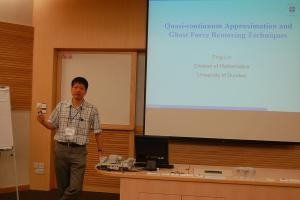Mass Conservative and Energy Stable Finite Difference Methods for the Quasi-incompressible Navier-Stokes-Cahn-Hilliard System: Primitive Variable and Projection-Type Schemes
Speaker
Prof. Ping Lin
University of Dundee and University of Science and Technology Beijing
Abstract

In this talk we describe two fully mass conservative, energy stable, finite difference methods on a staggered grid for the quasi-incompressible Navier-Stokes-Cahn-Hilliard (q-NSCH) system governing a binary incompressible fluid flow with variable density and viscosity. Both methods, namely the primitive method (finite difference method in the primitive variable formulation) and the projection method (finite difference method in a projection-type formulation), are so designed that the mass of the binary fluid is preserved, and the energy of the system equations is always non-increasing in time at the fully discrete level. We also present an efficient, practical nonlinear multigrid method - comprised of a standard FAS method for the Cahn-Hilliard equation, and a method based on the Vanka-type smoothing strategy for the Navier-Stokes equation - for solving these equations. We test the scheme in the context of Capillary Waves, rising droplets and Rayleigh-Taylor instability. Quantitative comparisons are made with existing analytical solutions or previous numerical results that validate the accuracy of our numerical schemes. Moreover, in all cases, mass of the single component and the binary fluid was conserved up to 10^{－8} and energy decreases in time. This is a joint work with Zhenlin Guo, John Lowengrub and Steven Wise.